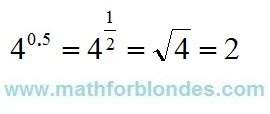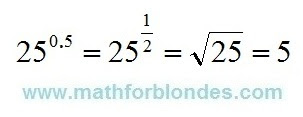## Pages

Showing posts with label power. Show all posts
Showing posts with label power. Show all posts

## 10.30.2021

### 2 to the power zero five

How much is 2 to the power zero five? A trick question, like everything mathematicians come up with for us. In short, transformations look like this.2 to the power zero five

Now let's take a closer look at the logic of mathematicians. Raising a number to an integer power is the multiplication of these numbers. Raising a number to a fractional power is extracting the root of a number, with the number in the denominator showing which root to find. But the fraction itself can be written both in the form of a decimal fraction, and in the form of a regular fraction. We put all this in a pot, mix thoroughly and the mathematical puzzle for the textbook is ready.

You need to solve this puzzle in the following order. We convert the decimal fraction zero five into a regular fraction and get one second. Now we can write the number 2 with a fractional exponent as the square root of two and calculate its value.

To consolidate knowledge, consider two more simple examples.4 to the power zero five

4 to the power zero five is the same as 4 to the one-second power. Four to the power of one second is the square root of 4. Taking the square root of four gives two.25 to the power zero five

After considering the two previous examples, it will be very easy to calculate 25 to the power zero five. As real shamans, we turn zero five into one second, extract the square root of 25 and get the desired result - the root of twenty-five is five.

Why all these dances of shamans with tambourines? There are two sides to this math medal. Front side - mathematicians teach us to use mathematics. The other side of the coin is that if mathematicians simply and clearly express their thoughts in the language of mathematics, then they will not seem so smart to us, and we ourselves will not look such fools.